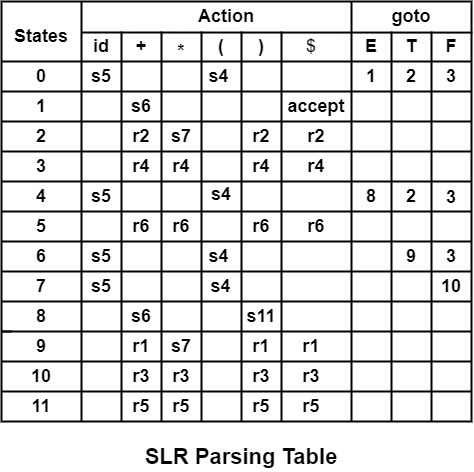# How to Fill the Entries in Parsing Table?

A parser is the second phase of compilation. The parser takes as its input tokens generated from the previous phase, i.e., the Lexical Analyzer phase, and groups them in such a way that their syntax can be recognized.

For example,

Consider I0

I0 − E′ → âˆ™ E

E → âˆ™ E + T

E → âˆ™ T

T → âˆ™ T ∗ F

T → âˆ™ F

F → âˆ™ (E)

F → âˆ™ id

Filling of Shifting Entries

Applying Rule (2a) of the algorithm of construction of SLR Parsing Table on a set of a production of I0. Rule (2a) will be applied only when there is a terminal symbol after the dot. Consider F → âˆ™ (E)

Compare A → α âˆ™ a β with F → âˆ™ (E)

 F → ε âˆ™ ( E ) A → α . a B

∴ A = F, α = ε, a = (, β = E)

Applying Rule, goto (Ii, a) = Ij

âˆµ     goto (I0, ( ) = I4

∴    Action [0, ( ] = Shift 4

∴ Write s4 in front of Row 0 and column (

Similarly, compare F → âˆ™ id

Applying Rule (2a)

Compare A → α âˆ™ a β with F → âˆ™ id

 F → ε âˆ™ id E A → α . a B

∴ A = F, α = ε, a = id, β = ε

∴ goto (I0, id) = I5

∴ Action [0, id] = shift 5

Write s5 in front of Row 0 and column id

Similarly, the complete table will be filled with shift actions by processing all states from I0 to I11 in a similar manner.

Filling of Reduce Entries (r) − For filling reduce (r) entries, we have to apply rule (2b) of the construction of the SLR Parsing Table.

From I0 to I11 we have to see productions of the form A → α âˆ™

Consider

I2 − E → T âˆ™

T → T âˆ™ * F

Here, we have the production E → T âˆ™ of form A → α âˆ™ in which dot appears at last position. So, compare A → α âˆ™ with E → T âˆ™

 E → T âˆ™ A → α .

Applying Rule, we have to find FOLLOW (E)

FOLLOW (E) = { +, ), $} The production number of E → T in Question is (2) Write r2 in front of the row of state 2 and column of +,),$.

action [2, +] = r2, action [2, )] = r2, action [2, $] = r2. Here, r2 refers to reduce using production number 2 Similarly, consider another state I3 I3 − T → F âˆ™ Apply Rule (2b) Compare T → F âˆ™ with A → α âˆ™  T → F âˆ™ A → α . ∴ A = T, α = F FOLLOW (T) = { +,*, ),$}

Since T → F is production number (4) in Question

Write r4 in front of the row of state 3 and column of +,*, ), $. Action [3, +] = r4 Action [3,$] = r4

Action [3, )] = r4                  Action [3, )] = r4

Similarly, fill up the Action table by reduce (r) entries.

Filling of goto Entries − goto Table can be filled for the non-terminals using Rule (3) of construction of Parsing Table.

Since,             I1 = goto(I0, E)

Applying Rule (3)

goto[0, E] = 1

Write 1 in front of Row 0 and Column E.

Similarly,          goto (I0, T) = I2

Applying Rule (3)

goto [0, T] = 2

Write 2 in front of Row 0 and Column T.

Similarly, we can fill other entries in goto Table

Filling of "accept" entries

Applying Rule (2C), there is a production.

E′ → E âˆ™ in I1.

Therefore, write "accept" in front of Row 1 and Column $. ∴ action [1,$] = "accept. "

By filling all shift, reduce, accept, goto entries, we get the following SLR parsing table.

In table s means shift, r means to reduce.